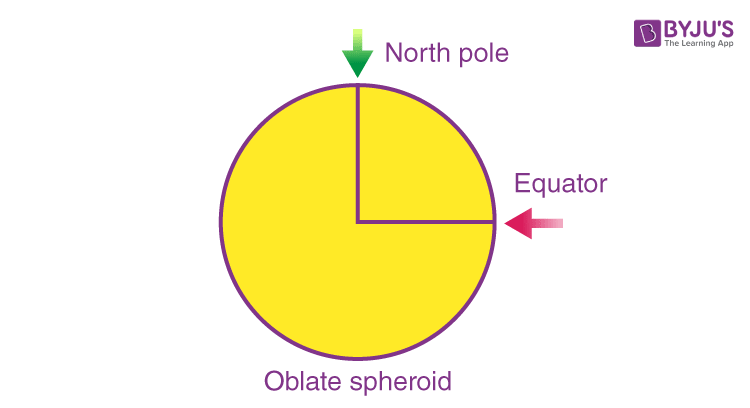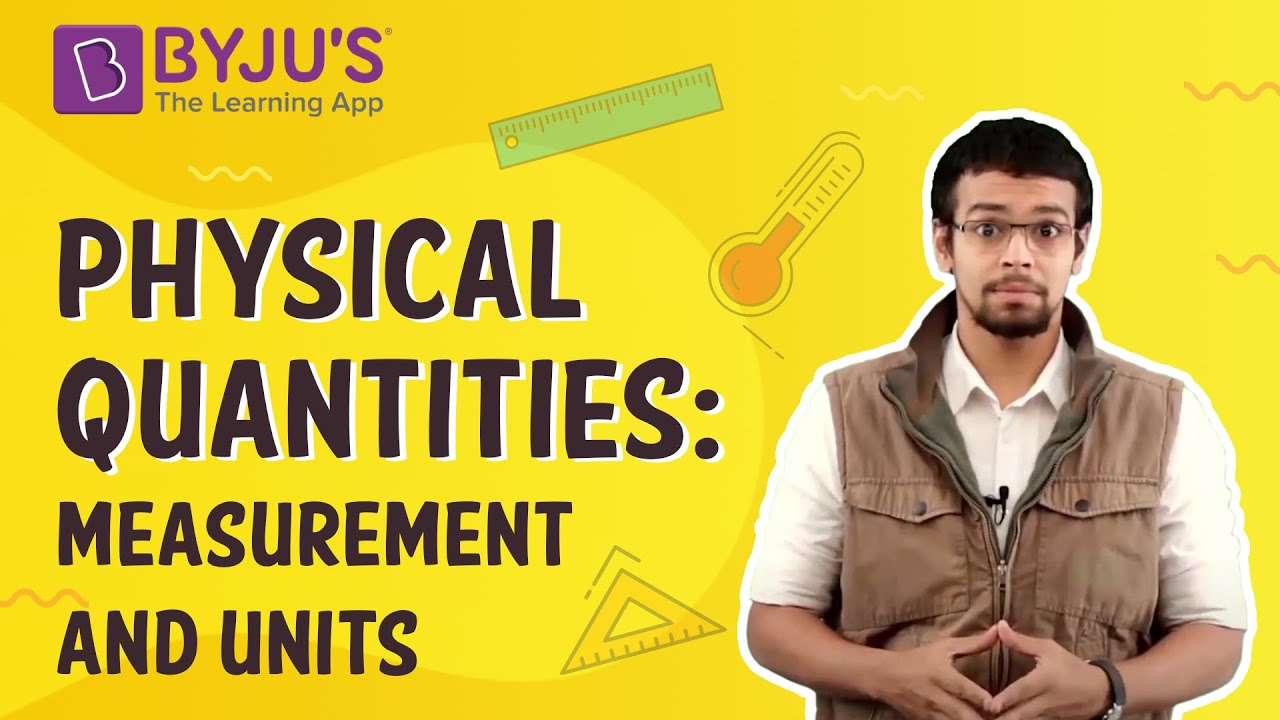Jet Set Go! All about Aeroplanes Jet Set Go! All about Aeroplanes

# Mass And Weight

The difference between mass and weight is one of the most frequently asked questions. Some students often use the terms mass and weight interchangeably, which is completely wrong.In this article, we are going to learn what mass and weight are and what are the key differences between them.

## What is Mass?

It is one of the fundamental quantities in Physics and the most basic property of matter. We can define mass as the measure of the amount of matter in a body. The SI unit of mass is Kilogram (kg).

Note: The mass of a body does not change at any time. Only for certain extreme cases when a huge amount of energy is given or taken from a body. For example: in a nuclear reaction, tiny amount of matter is converted into a huge amount of energy, this reduces the mass of the substance.

 More On Mass Measurement of Mass Center of Mass

## What is Weight?

It is the measure of the force of gravity acting on a body.

The formula for weight is given by:

w = mg

As weight is a force its SI unit is also the same as that of force, SI unit of weight is Newton (N). Looking at the expression of weight we see that it depends on mass and the acceleration due to gravity, the mass may not change but the acceleration due to gravity does change from place to place. To understand this concept let’s take this example,

Shape of the earth is not completely spherical, but an oblate spheroid, therefore a person standing at the equator is far away from the center of the earth than a person standing at the north pole, as acceleration due to gravity is proportional to the inverse of the square of the distance between two objects, a person standing at the north pole would experience more weight as he is closer to the center of the earth than a person standing at the equator.

### How is Weight Measured?

Following is the formula of a body which has a mass m and weight of magnitude w:

 w = mg

So it can be said that the weight of an object is directly proportional to its mass.## What is the Difference between Mass and Weight?

### Weight

Mass can never be zero. Weight can be zero. As in space if no gravity acts upon an object, its weight becomes zero.
Mass is a scalar quantity. It has magnitude. Weight is a vector quantity. It has magnitude and is directed toward the center of the Earth or other gravity well.
Mass is commonly measured in kilograms and grams. Weight is commonly measured in Newtons.
Mass doesn’t change according to location. Weight varies according to location.
The mass may be measured using an ordinary balance. Weight is measured using a spring balance.

### What is Weightlessness?

Have you ever been in an elevator? Noticed how you feel like your weight is been reduced while the elevator goes down? That is because the weight you feel is the perceived weight or “effective weight”, this is the equal and opposite force the floor exerts on you due to your weight. Now if we remove the floor and let you fall freely, there is nothing to exert a force on you and hence you will feel weightless even though there is acceleration due to gravity and mass. This is because the effective weight is equal to zero. Now, let’s come back to our elevator when the elevator is going down it is actually moving in the direction of the gravity, hence reducing net acceleration due to gravity, thereby reducing your weight.The same happens with astronauts in the international space station, as the space stations are orbiting the earth it is actually falling towards the earth indefinitely and everything in it is falling including the astronauts so the astronauts experience weightlessness and can float freely around. In all these scenarios the most important thing we have to notice is that weight can increase or decrease depending on the acceleration due to gravity but the mass remains unchanged.

## Relation Between Weight and Mass

Consider a body having large mass and large weight. Example of this situation is a large object which is hard to throw because the weight of this object is large.

Therefore, the relation between weight and mass can be derived with the help of Newton’s second law which explains that the free falling object has an acceleration “g” as the magnitude.

If an object with a mass of 1kg falls with an acceleration of 9.8 ms-2, then the magnitude of the force is given as :
F = ma
= (1kg)(9.8ms-2)
= (9.8 kg.ms-2)
= 9.8 N

Therefore, it can be concluded that the relation between weight and mass of an object with 1kg mass will have a weight of 9.8N.

## Frequently Asked Questions – FAQs

### What is meant by mass?

Mass can be stated as the measure of the quantity of matter in an object. The SI unit of mass is the kilogram (kg).

### What is meant by weight?

Weight is the measure of the force of gravity acting on a body.The formula for weight is given by:

w = mg

### What is the relationship between mass and weight?

Technically, weight is the mass of a body multiplied by the acceleration due to gravity.

### What is weightlessness?

Weightlessness is a condition of free-fall in which the gravity is cancelled by the inertial force arising from orbital flight or other gravity-negating situations. It is experienced due to the absence of the feeling of weight. It happens in the lack of any contact forces acting on bodies (human body).

### State Newton’s second law of motion.

This law is a quantitative explanation of the changes that a force can generate in a body’s motion. The second law states that when a body encounters a force, the time rate of change of the body’s momentum is equivalent to the force. The momentum of an object is the product of the object’s mass and velocity.

Stay tuned to BYJU’S and Fall in Love with Learning!

## Watch the video to find out what are base measurements?Test your Knowledge on Mass And Weight